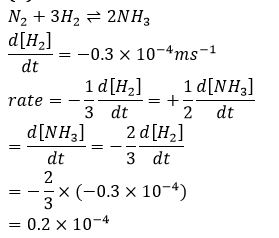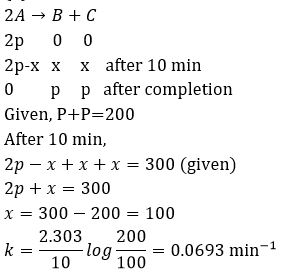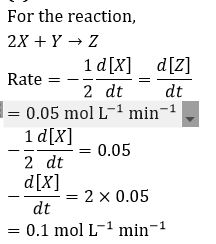## .question { margin-bottom: 20px; border-bottom: 1px solid #ddd; } .ques { font-size: 15px; font-weight: bold; line-height: 150%; } .q-options { padding: 0 !important; list-style: none; margin: 15px 0 !important; } .opt { font-size: 14px; font-weight: normal; } .answer { color: blue; display: none; padding-bottom: 15px; line-height: 150%; } .opt-right.active{ background: #a3ff91; font-size: 19px; line-height: 150%; border: 1px solid ##a3ff91; -webkit-border-radius: 5px; -moz-border-radius: 5px; border-radius: 5px; margin: 10px 0; } .mcq-info { margin: 20px 0 40px; color: #060553; line-height: 150%; } .timer{ overflow: hidden; margin: 20px 0; background: #f7f7f7; padding: 10px; border: 1px solid #eee; border-radius: 3px; } .start-btn, .time-btn, .stop-btn, #reset-start{ border: 1px solid #777; -o-border-radius: 2px; -ms-border-radius: 2px; -webkit-border-radius: 2px; border-radius: 2px; color: #fff; padding: 7px 15px; width: auto; height: auto; line-height: 150%; background: #d12F2F; cursor: pointer; margin-right: 10px; } #reset-start{ background: #0E2230; color: #fff; border-color: #0E2230; display: none; } .start-btn:hover, .time-btn:hover, .stop-btn:hover{ background: #0E2230; color: #fff; border-color: #0E2230; } #reset-start:hover{ background: #d12F2F; color: #fff; border-color: #d12F2F; } @media screen and (max-width: 767px){ .ques, .answer, .mcq-info, .opt{ font-size: 13px; } } Q1. Give relation between half reaction time (t_(1/2))and initial concentration of reactant for (n-1)order reaction.  t_(1/2)∝[R]_0  t_(1/2)∝[R]_0^(n-2)  t_(1/2)∝[R]_0^(n+1)  t_(1/2)∝[R]_0^(2-n) Solution Relation between (t_(1/2))and initial concentration of reactant for (n-1)order reaction t_(1/2)∝[R]_0^(2-n) Q2.For the reaction N_2+3H_2⇌2NH_3 The rate of change of concentration for hydrogen is 0.3×〖10〗^(-4) Ms^(-1) The rates of change of concentration of ammonia is  -0.2×〖10〗^(-4)  0.2×〖10〗^(-4)  0.1×〖10〗^(-4)  0.3×〖10〗^(-4) SolutionQ3.  For the reaction system 2NO(g)+O_2 (g)→2NO_2 (g) if the volume of the reaction vessel is reduced to one-third of its original volume, what will be the order of the reaction?   Diminished to one fourth of its initial value  Diminished to one twenty seven of its initial value  Increase to four times of its initial value  Increase to twenty seven times of its initial value SolutionQ4. The rate constant of nth order has units:  litre^(1-n) mol^(1-n) sec^(-1)⁡  mol^(n-1) litre^(n-1) sec^(-1)⁡  None of these  mol^(1-n) litre^(n-1) sec^(-1)⁡ Solution For nth order; unit of rate constant may be derived by K=rate/[reactant]^n Q5.In the reaction; A+2B⟶3C+D which of the following expressions does not describe changes in the concentration of various species as a function of time?  (2d[B])/dt=(d[A])/dt  (3d[B])/dt=(-2d[C])/dt  (3d[D])/dt=(d[C])/dt  (d[C])/dt=(-3d[A])/dt Solution  For the given reaction, (-d[A])/dt=-1/2 d[B]/dt=1/3 d[C]/dt=(d[D])/dt Q6. The velocity constant of a reaction at 290 K was found to be 3.2×〖10〗^(-3) s^(-1). When the temperature is raised to 310 K, it will be about  6.4×〖10〗^(-3)  1.28×〖10〗^(-2) 9.6×〖10〗^(-3)  3.2×〖10〗^(-4) Solution Rate constant almost gets doubled by the increase of 10℃ in temperature. Hence, the rate constant at 310 K will be =3.2×〖10〗^(-3)×(2)^2 ( ∵increase in temperature=20 K) =1.28×〖10〗^(-2) J^(-1) Q7.The term (-dc)/dt in a rate equation refers to  The velocity constant of the reaction  The change in concentration of the reactant  TThe decrease in concentration of the reactant with time  The concentration of the reactant Solution dc/dt represent the change in concentration of reactant with time. As, in a reaction, concentration of reactant always decrease with time hence, rate of reaction is represented as (-dc)/dt. Q8.The following data were obtained the first order decomposition of 2 A(g)→B(g)+C(S) at a constant volume and at a particular temperature SN Time Total pressure in Pascal 1 At the end of 10 min 300 2 After completion 200 The rate constant in min┴(-1)⁡〖is 〗  69.3  0.0693  6.93  6.93×〖10〗^(-4) SolutionQ9.For a chemical reaction 2X+Y→Z, the rate of appearance of Z is 0.05 mol L^(-1). The rate of disappearance of X will be  0.05 mol L^(-1) h^(-1)  0.05 mol L^(-1) min^(-1)  0.25 mol L^(-1) min^(-1)  0.1 mol L^(-1) min^(-1) SolutionQ10. An endothermic reaction A→B has an activation energy as x kg mol^(-1) of A. If energy change of the reaction is y kJ, the activation energy of the reverse reactions is  x+y  x-y  y-x -x Solutionvar answers = ["D","B","D","D","A","B","C","B","D","B"]; var tot = answers.length; function getCheckedValue( radioName ){ var radios = document.getElementsByName( radioName ); //console.log(radios) for (var y=0; y < radios.length; y++){ if (radios[y].checked) return radios[y].value; } } function getScore(){ var score = 0; for (var i=0; i<tot; i++){ var answerVal = getCheckedValue('question'+i) //console.log(answerVal, answers[i]) if (typeof answerVal != undefined && answerVal == answers[i]) score += 1; } return score; } function returnScore(){ alert('Your score is' +getScore()+"/"+tot); } var q, m, s; function quizCount(){ if(!q){ console.log('mobile2'); m = 1, s = 0; } if(q){ clearTimeout(q); } m = 10, s = 0; \$('#timer').val(m + ":" + s); s = s-1; console.log(s) q = setTimeout("counter()", 1000); if(s<1){ m = m-1; console.log(m) s = 59; } } function counter(){ console.log(m); \$('#timer').val(m + ":" + s); s = s-1; console.log(s) q = setTimeout("counter()", 1000); if(s<1){ m = m-1; console.log(m) s = 59; } if(m<0){ quizStop(); } } function quizStop(){ clearTimeout(q); q= null; m = null; s = null; document.getElementById('timer').value= 'Quiz Finished'; alert("Your score is "+ getScore() +"/"+ tot); \$('.answer').slideDown(); \$('.opt-right').addClass('active'); \$('.start-btn, #reset').hide(); \$('#reset-start').show(); } function quizReset(){ clearTimeout(q); document.getElementById('timer').reset(); } \$(document).ready(function(){ // var a = \$('.question .q-options li .rad').length; \$('.submit').click(function(){ returnScore(); \$('.opt-right').addClass('active'); \$('.answer').slideDown(); }); \$('#reset').click(function(){ quizReset(); }); \$('#reset-start').click(function(){ \$('.opt-right').removeClass('active'); \$('.answer').slideUp(); \$('.start-btn, #reset').show(); \$('#reset-start').hide(); \$('.rad').prop('checked', false); }); }); { "@context": "https://schema.org/", "@type": "Quiz", "typicalAgeRange": "7-12", //ADD THE REQUIRED AGE RANGE "educationalLevel": "intermediate", //ADD THE DIFFICULTY "assesses": "chemical kinetics", //ADD THE TOPIC NAME "educationalAlignment": [ {"@type": "AlignmentObject", "alignmentType": "educationalSubject", "targetName": "Science" } //ADD THE TOPIC NAME IN &#8220;targetName&#8221; BLOCK,ADD &#8216;,&#8217;(COMMA) AND REPEAT THE HIGHLIGHTED //BLOCK FOR ADDING MORE TOPICS ], "name": "Quiz about chemical kinetics",//ADD THE //QUESTION TOPIC "about": { "@type": "Thing", "name": "chemical kinetics"//ADD THE QUESTION TOPIC }, "hasPart": [ { "@type": "Question", "eduQuestionType": "Multiple choice", //Question type "learningResourceType": "Practice problem", "name": "chemical kinetics-1",//ADD QUESTION NAME "text": "\$\$Give relation between half reaction time (t_(1/2))and initial concentration of reactant for (n-1)order reaction.\$\$",//ADD THE QUESTION TEXT "comment": { "@type": "Comment", "Text": "This is a hint of question"//ADD HINT FOR QUESTIONS }, "encodingFormat": "text/markdown", "suggestedAnswer": [ //HERE PUT THE WRONG ANSWERS/OPTIONS { "@type": "Answer", "position": 0,//OPTION POSITION 0,1,2,3 "encodingFormat": "text/markdown", "text": "\$\$t_(1/2)&#8733;[R]_0\$\$",//ADD THE ANSWER "comment": { "@type": "Comment", "text": "This is a hint" //COMMENT FOR ANSWER } }, { "@type": "Answer", "position": 1, "encodingFormat": "text/markdown", "text": "\$\$t_(1/2)&#8733;[R]_0^(n-2)\$\$", //ADD THE ANSWER "comment": { "@type": "Comment", "text": "This is a hint"//COMMENT FOR ANSWER } }, { "@type": "Answer", "position": 2, "encodingFormat": "text/markdown", "text": "\$\$ t_(1/2)&#8733;[R]_0^(n+1)\$\$",//ADD THE ANSWER "comment": { "@type": "Comment", "text": "This is a hint"//ADD COMMENT FOR ANSWER } } ], "acceptedAnswer": { //HERE PUT THE CORRECT ANSWER "@type": "Answer", "position": 3, "encodingFormat": "text/markdown", "text": "\$\$ t_(1/2)&#8733;[R]_0^(2-n)\$\$",//ADD THE ANSWER BETWEEN &#8220; &#8220; "comment": { "@type": "Comment", "text": "This is a hint"//ADD COMMENT FOR ANSWER } } }, { "@type": "Question", "eduQuestionType": "Multiple choice", //Question type "learningResourceType": "Practice problem", "name": "chemical kinetics-2",//ADD QUESTION NAME "text": "\$\$For the reaction N_2+3H_2&#8652;2NH_3 The rate of change of concentration for hydrogen is 0.3&#215;&#12310;10&#12311;^(-4) Ms^(-1) The rates of change of concentration of ammonia is\$\$",//ADD THE QUESTION TEXT "comment": { "@type": "Comment", "Text": "This is a hint of question"//ADD HINT FOR QUESTIONS }, "encodingFormat": "text/markdown", "suggestedAnswer": [ //HERE PUT THE WRONG ANSWERS/OPTIONS { "@type": "Answer", "position": 0,//OPTION POSITION 0,1,2,3 "encodingFormat": "text/markdown", "text": "\$\$-0.2&#215;&#12310;10&#12311;^(-4)\$\$",//ADD THE ANSWER "comment": { "@type": "Comment", "text": "This is a hint" //COMMENT FOR ANSWER } }, { "@type": "Answer", "position": 2, "encodingFormat": "text/markdown", "text": "\$\$0.1&#215;&#12310;10&#12311;^(-4)\$\$", //ADD THE ANSWER "comment": { "@type": "Comment", "text": "This is a hint"//COMMENT FOR ANSWER } }, { "@type": "Answer", "position": 3, "encodingFormat": "text/markdown", "text": "\$\$ 0.3&#215;&#12310;10&#12311;^(-4)\$\$",//ADD THE ANSWER "comment": { "@type": "Comment", "text": "This is a hint"//ADD COMMENT FOR ANSWER } } ], "acceptedAnswer": { //HERE PUT THE CORRECT ANSWER "@type": "Answer", "position": 1, "encodingFormat": "text/markdown", "text": "\$\$ 0.2&#215;&#12310;10&#12311;^(-4)\$\$",//ADD THE ANSWER BETWEEN &#8220; &#8220; "comment": { "@type": "Comment", "text": "This is a hint"//ADD COMMENT FOR ANSWER } } }, { "@type": "Question", "eduQuestionType": "Multiple choice", //Question type "learningResourceType": "Practice problem", "name": "chemical kinetics-3",//ADD QUESTION NAME "text": " \$\$ For the reaction system 2NO(g)+O_2 (g)&#8594;2NO_2 (g) if the volume of the reaction vessel is reduced to one-third of its original volume, what will be the order of the reaction? \$\$",//ADD THE QUESTION TEXT "comment": { "@type": "Comment", "Text": "This is a hint of question"//ADD HINT FOR QUESTIONS }, "encodingFormat": "text/markdown", "suggestedAnswer": [ //HERE PUT THE WRONG ANSWERS/OPTIONS { "@type": "Answer", "position": 0,//OPTION POSITION 0,1,2,3 "encodingFormat": "text/markdown", "text": "Diminished to one fourth of its initial value",//ADD THE ANSWER "comment": { "@type": "Comment", "text": "This is a hint" //COMMENT FOR ANSWER } }, { "@type": "Answer", "position": 1, "encodingFormat": "text/markdown", "text": "Diminished to one twenty seven of its initial value", //ADD THE ANSWER "comment": { "@type": "Comment", "text": "This is a hint"//COMMENT FOR ANSWER } }, { "@type": "Answer", "position": 2, "encodingFormat": "text/markdown", "text": "Increase to four times of its initial value",//ADD THE ANSWER "comment": { "@type": "Comment", "text": "This is a hint"//ADD COMMENT FOR ANSWER } } ], "acceptedAnswer": { //HERE PUT THE CORRECT ANSWER "@type": "Answer", "position": 3, "encodingFormat": "text/markdown", "text": " Increase to twenty seven times of its initial value",//ADD THE ANSWER BETWEEN &#8220; &#8220; "comment": { "@type": "Comment", "text": "This is a hint"//ADD COMMENT FOR ANSWER } } }, { "@type": "Question", "eduQuestionType": "Multiple choice", //Question type "learningResourceType": "Practice problem", "name": "chemical kinetics-4",//ADD QUESTION NAME "text": "The rate constant of nth order has units:",//ADD THE QUESTION TEXT "comment": { "@type": "Comment", "Text": "This is a hint of question"//ADD HINT FOR QUESTIONS }, "encodingFormat": "text/markdown", "suggestedAnswer": [ //HERE PUT THE WRONG ANSWERS/OPTIONS { "@type": "Answer", "position": 0,//OPTION POSITION 0,1,2,3 "encodingFormat": "text/markdown", "text": "\$\$ litre^(1-n) mol^(1-n) sec^(-1)&#8289;\$\$",//ADD THE ANSWER "comment": { "@type": "Comment", "text": "This is a hint" //COMMENT FOR ANSWER } }, { "@type": "Answer", "position": 1, "encodingFormat": "text/markdown", "text": "\$\$mol^(n-1) litre^(n-1) sec^(-1)&#8289;\$\$", //ADD THE ANSWER "comment": { "@type": "Comment", "text": "This is a hint"//COMMENT FOR ANSWER } }, { "@type": "Answer", "position": 2, "encodingFormat": "text/markdown", "text": "None of these",//ADD THE ANSWER "comment": { "@type": "Comment", "text": "This is a hint"//ADD COMMENT FOR ANSWER } } ], "acceptedAnswer": { //HERE PUT THE CORRECT ANSWER "@type": "Answer", "position": 3, "encodingFormat": "text/markdown", "text": "\$\$ mol^(1-n) litre^(n-1) sec^(-1)&#8289;\$\$",//ADD THE ANSWER BETWEEN &#8220; &#8220; "comment": { "@type": "Comment", "text": "This is a hint"//ADD COMMENT FOR ANSWER } } }, { "@type": "Question", "eduQuestionType": "Multiple choice", //Question type "learningResourceType": "Practice problem", "name": "chemical kinetics-5",//ADD QUESTION NAME "text": "\$\$In the reaction; A+2B&#10230;3C+D which of the following expressions does not describe changes in the concentration of various species as a function of time?\$\$",//ADD THE QUESTION TEXT "comment": { "@type": "Comment", "Text": "This is a hint of question"//ADD HINT FOR QUESTIONS }, "encodingFormat": "text/markdown", "suggestedAnswer": [ //HERE PUT THE WRONG ANSWERS/OPTIONS { "@type": "Answer", "position": 1,//OPTION POSITION 0,1,2,3 "encodingFormat": "text/markdown", "text": "\$\$(3d[B])/dt=(-2d[C])/dt\$\$",//ADD THE ANSWER "comment": { "@type": "Comment", "text": "This is a hint" //COMMENT FOR ANSWER } }, { "@type": "Answer", "position": 2, "encodingFormat": "text/markdown", "text": "\$\$(3d[D])/dt=(d[C])/dt\$\$", //ADD THE ANSWER "comment": { "@type": "Comment", "text": "This is a hint"//COMMENT FOR ANSWER } }, { "@type": "Answer", "position": 3, "encodingFormat": "text/markdown", "text": "\$\$(d[C])/dt=(-3d[A])/dt\$\$",//ADD THE ANSWER "comment": { "@type": "Comment", "text": "This is a hint"//ADD COMMENT FOR ANSWER } } ], "acceptedAnswer": { //HERE PUT THE CORRECT ANSWER "@type": "Answer", "position": 0, "encodingFormat": "text/markdown", "text": "\$\$(2d[B])/dt=(d[A])/dt\$\$",//ADD THE ANSWER BETWEEN &#8220; &#8220; "comment": { "@type": "Comment", "text": "This is a hint"//ADD COMMENT FOR ANSWER } } }, { "@type": "Question", "eduQuestionType": "Multiple choice", //Question type "learningResourceType": "Practice problem", "name": "chemical kinetics-6",//ADD QUESTION NAME "text": "\$\$The velocity constant of a reaction at 290 K was found to be 3.2&#215;&#12310;10&#12311;^(-3) s^(-1). When the temperature is raised to 310 K, it will be about\$\$",//ADD THE QUESTION TEXT "comment": { "@type": "Comment", "Text": "This is a hint of question"//ADD HINT FOR QUESTIONS }, "encodingFormat": "text/markdown", "suggestedAnswer": [ //HERE PUT THE WRONG ANSWERS/OPTIONS { "@type": "Answer", "position": 0,//OPTION POSITION 0,1,2,3 "encodingFormat": "text/markdown", "text": "\$\$ 6.4&#215;&#12310;10&#12311;^(-3)\$\$",//ADD THE ANSWER "comment": { "@type": "Comment", "text": "This is a hint" //COMMENT FOR ANSWER } }, { "@type": "Answer", "position": 2, "encodingFormat": "text/markdown", "text": "\$\$9.6&#215;&#12310;10&#12311;^(-3)\$\$", //ADD THE ANSWER "comment": { "@type": "Comment", "text": "This is a hint"//COMMENT FOR ANSWER } }, { "@type": "Answer", "position": 3, "encodingFormat": "text/markdown", "text": "\$\$ 3.2&#215;&#12310;10&#12311;^(-4)\$\$",//ADD THE ANSWER "comment": { "@type": "Comment", "text": "This is a hint"//ADD COMMENT FOR ANSWER } } ], "acceptedAnswer": { //HERE PUT THE CORRECT ANSWER "@type": "Answer", "position": 1, "encodingFormat": "text/markdown", "text": "\$\$1.28&#215;&#12310;10&#12311;^(-2)\$\$",//ADD THE ANSWER BETWEEN &#8220; &#8220; "comment": { "@type": "Comment", "text": "This is a hint"//ADD COMMENT FOR ANSWER } } }, { "@type": "Question", "eduQuestionType": "Multiple choice", //Question type "learningResourceType": "Practice problem", "name": "chemical kinetics-7",//ADD QUESTION NAME "text": "\$\$The term (-dc)/dt in a rate equation refers to \$\$",//ADD THE QUESTION TEXT "comment": { "@type": "Comment", "Text": "This is a hint of question"//ADD HINT FOR QUESTIONS }, "encodingFormat": "text/markdown", "suggestedAnswer": [ //HERE PUT THE WRONG ANSWERS/OPTIONS { "@type": "Answer", "position": 0,//OPTION POSITION 0,1,2,3 "encodingFormat": "text/markdown", "text": "The velocity constant of the reaction",//ADD THE ANSWER "comment": { "@type": "Comment", "text": "This is a hint" //COMMENT FOR ANSWER } }, { "@type": "Answer", "position": 1, "encodingFormat": "text/markdown", "text": "The change in concentration of the reactant", //ADD THE ANSWER "comment": { "@type": "Comment", "text": "This is a hint"//COMMENT FOR ANSWER } }, { "@type": "Answer", "position": 3, "encodingFormat": "text/markdown", "text": "The concentration of the reactant",//ADD THE ANSWER "comment": { "@type": "Comment", "text": "This is a hint"//ADD COMMENT FOR ANSWER } } ], "acceptedAnswer": { //HERE PUT THE CORRECT ANSWER "@type": "Answer", "position": 2, "encodingFormat": "text/markdown", "text": "TThe decrease in concentration of the reactant with time",//ADD THE ANSWER BETWEEN &#8220; &#8220; "comment": { "@type": "Comment", "text": "This is a hint"//ADD COMMENT FOR ANSWER } } }, { "@type": "Question", "eduQuestionType": "Multiple choice", //Question type "learningResourceType": "Practice problem", "name": "chemical kinetics-8",//ADD QUESTION NAME "text": "\$\$The following data were obtained the first order decomposition of 2 A(g)&#8594;B(g)+C(S) at a constant volume and at a particular temperature SN Time Total pressure in Pascal 1 At the end of 10 min 300 2 After completion 200 The rate constant in min&#9524;(-1)&#8289;&#12310;is &#12311;\$\$",//ADD THE QUESTION TEXT "comment": { "@type": "Comment", "Text": "This is a hint of question"//ADD HINT FOR QUESTIONS }, "encodingFormat": "text/markdown", "suggestedAnswer": [ //HERE PUT THE WRONG ANSWERS/OPTIONS { "@type": "Answer", "position": 0,//OPTION POSITION 0,1,2,3 "encodingFormat": "text/markdown", "text": "\$\$ 69.3\$\$",//ADD THE ANSWER "comment": { "@type": "Comment", "text": "This is a hint" //COMMENT FOR ANSWER } }, { "@type": "Answer", "position": 2, "encodingFormat": "text/markdown", "text": "\$\$ 6.93\$\$", //ADD THE ANSWER "comment": { "@type": "Comment", "text": "This is a hint"//COMMENT FOR ANSWER } }, { "@type": "Answer", "position": 3, "encodingFormat": "text/markdown", "text": "\$\$ 6.93&#215;&#12310;10&#12311;^(-4)\$\$",//ADD THE ANSWER "comment": { "@type": "Comment", "text": "This is a hint"//ADD COMMENT FOR ANSWER } } ], "acceptedAnswer": { //HERE PUT THE CORRECT ANSWER "@type": "Answer", "position": 1, "encodingFormat": "text/markdown", "text": "\$\$ 0.0693\$\$",//ADD THE ANSWER BETWEEN &#8220; &#8220; "comment": { "@type": "Comment", "text": "This is a hint"//ADD COMMENT FOR ANSWER } } }, { "@type": "Question", "eduQuestionType": "Multiple choice", //Question type "learningResourceType": "Practice problem", "name": "chemical kinetics-9",//ADD QUESTION NAME "text": "\$\$For a chemical reaction 2X+Y&#8594;Z, the rate of appearance of Z is 0.05 mol L^(-1). The rate of disappearance of X will be\$\$",//ADD THE QUESTION TEXT "comment": { "@type": "Comment", "Text": "This is a hint of question"//ADD HINT FOR QUESTIONS }, "encodingFormat": "text/markdown", "suggestedAnswer": [ //HERE PUT THE WRONG ANSWERS/OPTIONS { "@type": "Answer", "position": 0,//OPTION POSITION 0,1,2,3 "encodingFormat": "text/markdown", "text": "\$\$0.05 mol L^(-1) h^(-1)\$\$",//ADD THE ANSWER "comment": { "@type": "Comment", "text": "This is a hint" //COMMENT FOR ANSWER } }, { "@type": "Answer", "position": 1, "encodingFormat": "text/markdown", "text": "\$\$0.05 mol L^(-1) min^(-1)\$\$", //ADD THE ANSWER "comment": { "@type": "Comment", "text": "This is a hint"//COMMENT FOR ANSWER } }, { "@type": "Answer", "position": 2, "encodingFormat": "text/markdown", "text": "\$\$ 0.25 mol L^(-1) min^(-1)\$\$",//ADD THE ANSWER "comment": { "@type": "Comment", "text": "This is a hint"//ADD COMMENT FOR ANSWER } } ], "acceptedAnswer": { //HERE PUT THE CORRECT ANSWER "@type": "Answer", "position": 3, "encodingFormat": "text/markdown", "text": "\$\$ 0.1 mol L^(-1) min^(-1)\$\$",//ADD THE ANSWER BETWEEN &#8220; &#8220; "comment": { "@type": "Comment", "text": "This is a hint"//ADD COMMENT FOR ANSWER } } }, { "@type": "Question", "eduQuestionType": "Multiple choice", //Question type "learningResourceType": "Practice problem", "name": "chemical kinetics-10",//ADD QUESTION NAME "text": "\$\$An endothermic reaction A&#8594;B has an activation energy as x kg mol^(-1) of A. If energy change of the reaction is y kJ, the activation energy of the reverse reactions is\$\$",//ADD THE QUESTION TEXT "comment": { "@type": "Comment", "Text": "This is a hint of question"//ADD HINT FOR QUESTIONS }, "encodingFormat": "text/markdown", "suggestedAnswer": [ //HERE PUT THE WRONG ANSWERS/OPTIONS { "@type": "Answer", "position": 0,//OPTION POSITION 0,1,2,3 "encodingFormat": "text/markdown", "text": "\$\$ x+y\$\$",//ADD THE ANSWER "comment": { "@type": "Comment", "text": "This is a hint" //COMMENT FOR ANSWER } }, { "@type": "Answer", "position": 2, "encodingFormat": "text/markdown", "text": "\$\$ y-x\$\$", //ADD THE ANSWER "comment": { "@type": "Comment", "text": "This is a hint"//COMMENT FOR ANSWER } }, { "@type": "Answer", "position": 3, "encodingFormat": "text/markdown", "text": "\$\$-x\$\$",//ADD THE ANSWER "comment": { "@type": "Comment", "text": "This is a hint"//ADD COMMENT FOR ANSWER } } ], "acceptedAnswer": { //HERE PUT THE CORRECT ANSWER "@type": "Answer", "position": 1, "encodingFormat": "text/markdown", "text": "\$\$ x-y\$\$",//ADD THE ANSWER BETWEEN &#8220; &#8220; "comment": { "@type": "Comment", "text": "This is a hint"//ADD COMMENT FOR ANSWER } } } //ADD &#8216;,&#8217;(COMMA) AND REPEAT THE ABOVE HIGHLIGHTED PART,TO MARKUP MORE QUESTIONS ] }#### Written by: AUTHORNAME

AUTHORDESCRIPTION## Want to know more

Please fill in the details below:

## Latest NEET Articles\$type=three\$c=3\$author=hide\$comment=hide\$rm=hide\$date=hide\$snippet=hide

Name

ltr
item
BEST NEET COACHING CENTER | BEST IIT JEE COACHING INSTITUTE | BEST NEET, IIT JEE COACHING INSTITUTE: CHEMICAL KINETICS QUIZ-4
CHEMICAL KINETICS QUIZ-4